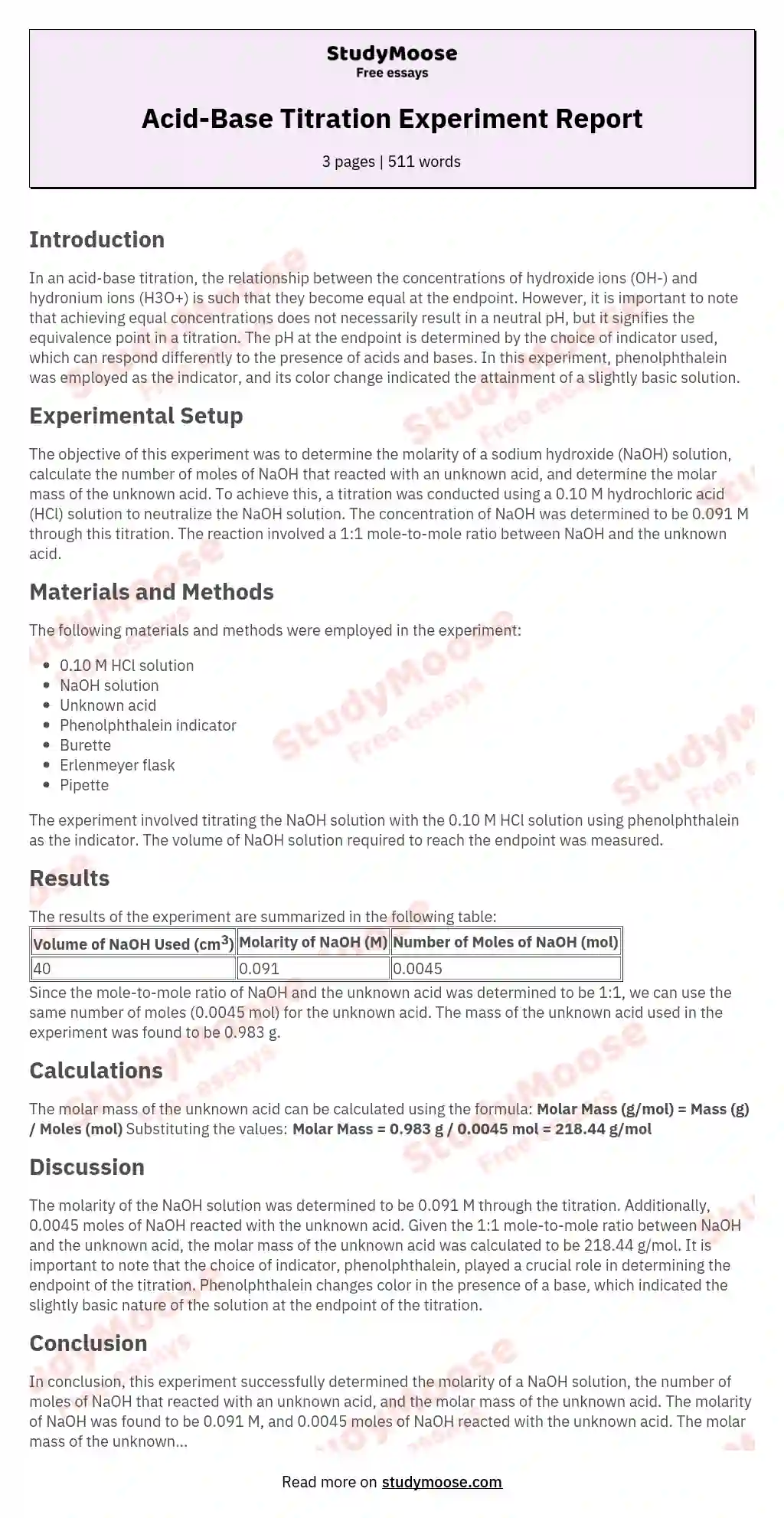# Acid-Base Titration Experiment Report

Categories: Titration

## Introduction

In an acid-base titration, the relationship between the concentrations of hydroxide ions (OH-) and hydronium ions (H3O+) is such that they become equal at the endpoint. However, it is important to note that achieving equal concentrations does not necessarily result in a neutral pH, but it signifies the equivalence point in a titration. The pH at the endpoint is determined by the choice of indicator used, which can respond differently to the presence of acids and bases. In this experiment, phenolphthalein was employed as the indicator, and its color change indicated the attainment of a slightly basic solution.

## Experimental Setup

The objective of this experiment was to determine the molarity of a sodium hydroxide (NaOH) solution, calculate the number of moles of NaOH that reacted with an unknown acid, and determine the molar mass of the unknown acid. To achieve this, a titration was conducted using a 0.10 M hydrochloric acid (HCl) solution to neutralize the NaOH solution. The concentration of NaOH was determined to be 0.

Get quality help nowRhizManVerified writer

Proficient in: Titration4.9 (247)

“ Rhizman is absolutely amazing at what he does . I highly recommend him if you need an assignment done ”+84 relevant experts are online

091 M through this titration. The reaction involved a 1:1 mole-to-mole ratio between NaOH and the unknown acid.

## Materials and Methods

The following materials and methods were employed in the experiment:

• 0.10 M HCl solution
• NaOH solution
• Unknown acid
• Phenolphthalein indicator
• Burette
• Pipette

The experiment involved titrating the NaOH solution with the 0.10 M HCl solution using phenolphthalein as the indicator. The volume of NaOH solution required to reach the endpoint was measured.

## Results

The results of the experiment are summarized in the following table:

Volume of NaOH Used (cm3) Molarity of NaOH (M) Number of Moles of NaOH (mol)
40 0.091 0.0045

Since the mole-to-mole ratio of NaOH and the unknown acid was determined to be 1:1, we can use the same number of moles (0.0045 mol) for the unknown acid. The mass of the unknown acid used in the experiment was found to be 0.983 g.

## Calculations

The molar mass of the unknown acid can be calculated using the formula:

Molar Mass (g/mol) = Mass (g) / Moles (mol)

Substituting the values:

Molar Mass = 0.983 g / 0.0045 mol = 218.44 g/mol

## Discussion

The molarity of the NaOH solution was determined to be 0.091 M through the titration. Additionally, 0.0045 moles of NaOH reacted with the unknown acid. Given the 1:1 mole-to-mole ratio between NaOH and the unknown acid, the molar mass of the unknown acid was calculated to be 218.44 g/mol.

It is important to note that the choice of indicator, phenolphthalein, played a crucial role in determining the endpoint of the titration. Phenolphthalein changes color in the presence of a base, which indicated the slightly basic nature of the solution at the endpoint of the titration.

## Conclusion

In conclusion, this experiment successfully determined the molarity of a NaOH solution, the number of moles of NaOH that reacted with an unknown acid, and the molar mass of the unknown acid. The molarity of NaOH was found to be 0.091 M, and 0.0045 moles of NaOH reacted with the unknown acid. The molar mass of the unknown acid was calculated to be 218.44 g/mol. This experiment demonstrated the principles of acid-base titration and the use of indicators in determining endpoints.